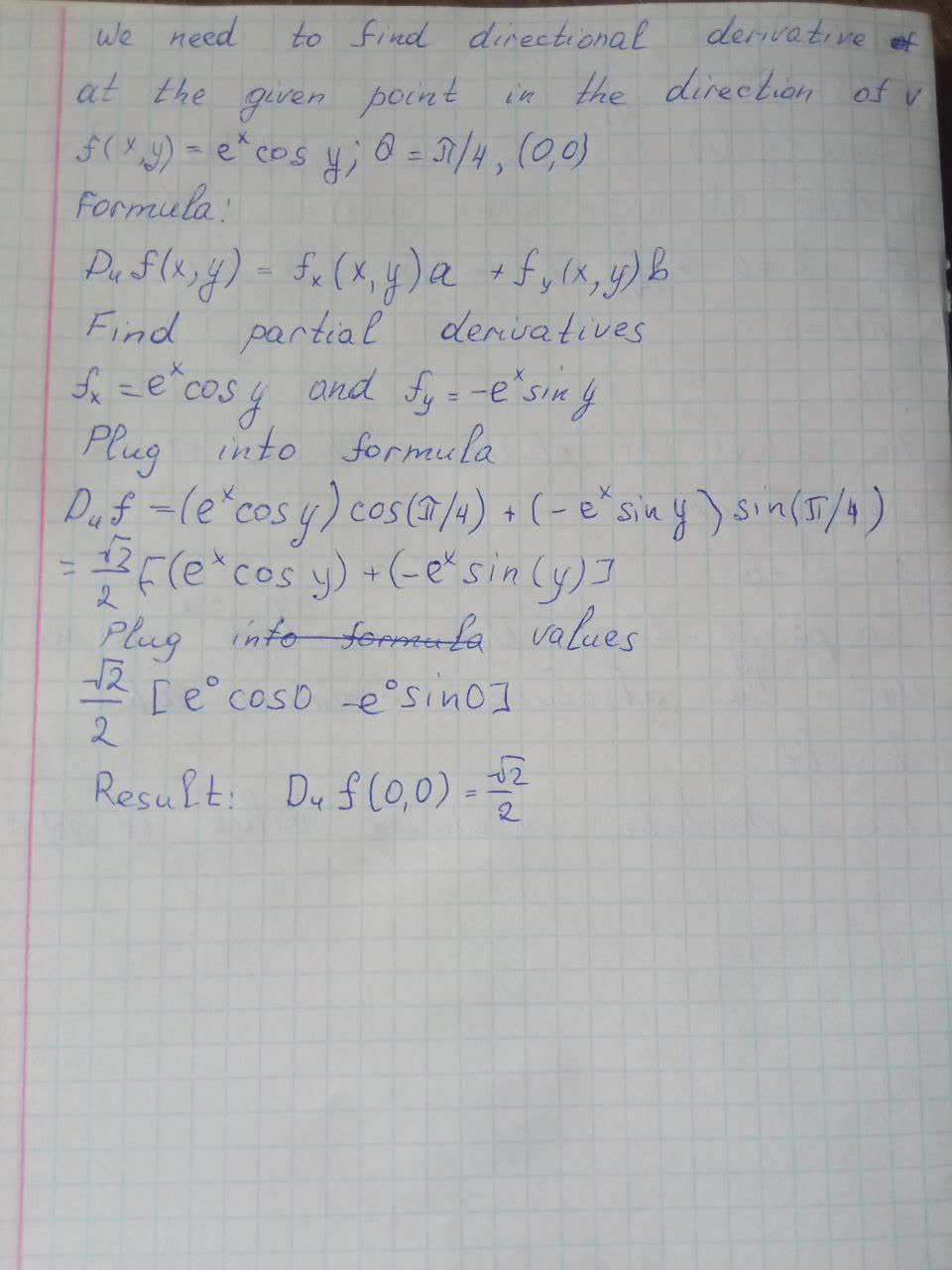# Find the directional derivative of f at the given point in the direction indicated by the angle \theta. f(x,y)=e^x\cos y,(0,0),\theta=\frac{\pi}{4}Sinead Mcgee 2021-05-19 Answered
Find the directional derivative of f at the given point in the direction indicated by the angle $\theta .f\left(x,y\right)={e}^{x}\mathrm{cos}y,\left(0,0\right),\theta =\frac{\pi }{4}$
You can still ask an expert for help

• Questions are typically answered in as fast as 30 minutes

Solve your problem for the price of one coffee

• Math expert for every subject
• Pay only if we can solve itDaphne Broadhurst
Photo below:###### Not exactly what you’re looking for?Jeffrey Jordon

We need to find directional derivative of at the given point in the direction of v

Formula:

${D}_{u}f\left(x,y\right)={f}_{x}\left(x,y\right)a+{f}_{y}\left(x,y\right)b$

Find partial derivatives

${f}_{x}={e}^{x}\mathrm{cos}y$ and ${f}_{y}=-{e}^{x}\mathrm{sin}y$

Plug into formula

${D}_{u}f=\left({e}^{x}\mathrm{cos}y\right)\mathrm{cos}\left(\pi /4\right)+\left(-{e}^{x}\mathrm{sin}y\right)\mathrm{sin}\left(\pi /4\right)$

$=\frac{\sqrt{2}}{2}\left[\left({e}^{x}\mathrm{cos}y\right)+\left(-{e}^{x}\mathrm{sin}\left(y\right)\right]$

Plug in values

$\frac{\sqrt{2}}{2}\left[{e}^{0}\mathrm{cos}0-{e}^{0}\mathrm{sin}0\right]$

Result: ${D}_{u}f\left(0,0\right)=\frac{\sqrt{2}}{2}$